V.6 No 1 S.B. Karavashkin and O.N. Karavashkina 1 Supplement 1: On the Green functionSupplement 1: On the Green function From: tadchem Date: Mon 13 March 2006 00:14 E-mail: "tadchem" Groups: sci.physics, alt.sci.physics, alt.sci.physics.new-theories, alt.astronomy, sci.physics.relativity Was there something about the chapter on Greens Functions that you missed? http://www.math.lsa.umich.edu/~vbooth/gf.pdf   Tom Davidson Richmond, VA Immediate reply Dear Tom, first of all thank you for this paper. Its style is fine and formulation strong. Just it shows the difference in our approach and the approach when solving with the Green function. We omitted this part, doing not wanting to dive into additional explanation, why the solutions yielded with the Green function are factually erroneous. Yes, really so, and kindly understand what I mean. To simplify, let us take the first problem of your paper. It is known that “the Green function has a simple physical meaning. It is the solution for a single source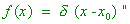(in the symbols of your paper) [M.L. Krasnov. Integral equations]. The Green function G (x, x0) will satisfy the solution if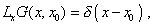(1)
 where Lx is the operator of a homogeneous differential equation related to the given heterogeneous equation. This is the equation which the Green function has factually to satisfy. And what they conventionally do? Both in your paper and usually, the solution is sought for the equation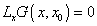(2)
 on the right and left from the point x0, then they join the solutions, basing on the artificial stipulation that the function is continuous at the very point. And your paper solves, proceeding from it. But (2) does not correspond to (1) namely at x0. At this point (1) becomes heterogeneous! And the Green function found with the conventional joining techniques is unable to account this basic feature, we can easily make it sure. Take any Green function determined so and substitute it to (1) at x - x0 . You will yield zero in the whole interval, including the point x0, not the delta-function. This is not a solution, as, when you affect the solution by the operator of differential equation L, it will turn the Green function to zero, while it has to give a delta-function; multiplying it into the right-part function of the equation and integrating, you have to yield the right part of the initial differential equation. Thus, it only seems that you seek the solution of heterogeneous differential equation. In fact, you find some kind of solution of the homogeneous equation. ;-) So the solutions found by our technique will generally differ from the numerical solutions yielded with the Green function. You can see from our material that we even have not this difficulty and yield analytic solutions without delta-function. And in many cases we yield them even not as a Fourier-expansion but analytically, which is basically impossible in case of Green function. And this last is shown in our paper. But perhaps you are right, we would have to express it in a detailed proof. Well, we will do the supplement to this paper and then tell you. I would hope that you will attentively analyse what I said, as I understand how unexpected and unusual is it for you. Best, Sergey Detailed reply As I promised, now I will try to substantiate my statement that it is incorrect to solve the problems using the Green function. Let us write the standard requirements to this function when seeking the solution for a trivial second-order differential equation of the kind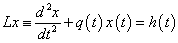(1)
 with the boundary conditions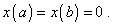(2)
 “Definition: We call the Green function G (t, s) of the boundary problem (1)- (2) the function of two variables determined in the square a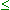t, sb so that 1) Lt G (t, s) = 0  with t < s and t > s, because G (t, s)   as the function of t at definite values of t and s satisfies the equation (1); 2) The function G (t, s)   satisfies the given boundary conditions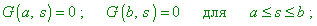(3)
 3) G (t, s)   is continuous at t = s: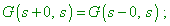(4)
 4) The derivative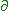G/t at the point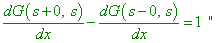(5)
 [1, p. 221]. These conditions have to provide the validity of equality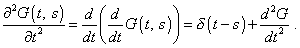(6)
 “So we may substitute the conditions 1) and 4) by the condition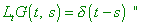(7)
 [1, p. 222]. This modified condition (7) is caused by the following aspect: “The solution of boundary condition (1)- (2) is given by the formula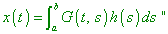(8)
 [1, p. 222]. With it, the chain of equalities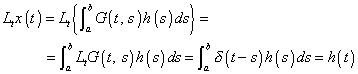(9)
 has to be true for (8). We see that (7) was directly used in (9). And in order (9) to be strongly true, it is necessary, (7) to be strongly true, too. Now let us analyse from this view the conventional technique to find the Green function that is shown, in particular, in the paper you sent me. Consider it on the example described in the above mentioned book by Krasnov , although he gives a standard technique used by other authors, with exception of only some trifles. Consider an equation of the kind (1)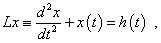(10)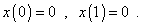(11)
 “Let us plot the Green function of the problem (10)- (11). The general solution of the homogeneous equation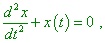(12)
 corresponding to (10) is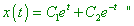(13)
 [1, p. 222]. But what concern has (12) to (7) which the Green function really has to satisfy strongly? Only that out of the point t = s the function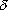(t - s) vanishes. But this is obviously insufficient for the strong correspondence. Factually we would have to seek the solution of the system of equations like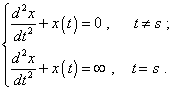(14)
 With it, we clearly can yield the solution of second equation neither in the explicit form nor as the series. And if we exclude the point t = s from the consideration, we in this way exclude the delta-function from the right part of equation and admit, the sought solution is not a solution, as with it the chain of equalities (9) gives not the right part of the initial differential equation but zero. And I can easily show it. Let us continue the analysis of Green function construction. “As the function G (t, s)  has to be the solution of this homogeneous equation at t < s and at t > s, let us represent it as follows: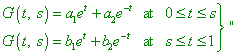(15)
 [1, p. 223]. Let us draw our attention to the boundaries of intervals in (15). In the premise we see strong inequalities, while in (15) they are substitute by those weak. What does it mean? This means that the mathematicians well understood when rewrote from book to book that (12) does not cover the crucially important point t = s. They also well understood that (15) would have to contain three equations and this third would have to describe just the solution at this point that, as I showed above, they cannot yield. So they silently substituted the symbols and it factually destroyed the whole construction of the Green function as a solution for the differential equation (10). Both the first and second equations in (15) satisfy the homogeneous equation (12); this means, at the point t = s the Green function also will satisfy this equation, not the second equation of (14), with all consequences for the basic proof defined by the chain of equalities (9). To the point, if we rigorously approach this stage of Green function construction, then changing the way to construct from the differential form, as it was described above, to that integral, as it was shown in your paper, changes, basically, nothing, as instead the expression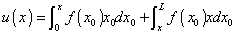(16)
 there would be the following: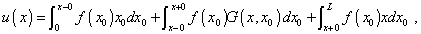(17)
 in which you never will be able to determine the middle integral directly either numerically, as in this integral the Green function has to be the solution just of the second equation of (14), or your Green function will not satisfy the fourth condition of definition, just as the above-considered function (15). In this last case it is easy to show it, as the cited author showed his construction till the end and showed this function in its final appearance: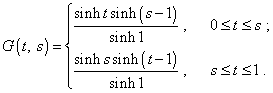(18)
 As we see from (18), the final system also consists of two solutions, and the point of our attention, t = s, is included to the domain of these solutions. The first derivative of (18) in time will have the following appearance: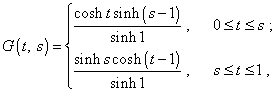(19)
 and the second derivative will replicate (18). Consequently, in the whole interval of existence, the solution will satisfy the related homogeneous differential equation (12), not (7) that determined the correspondence of Green function to the initial differential equation. If speaking of, what satisfies this solution yielded on the basis of such substitution of heterogeneous equation with the delta-function in the right part by the homogeneous equation, the conclusion follows from the very way to construct the Green function. Above we showed that if affecting by the operator onto the integral form of solution with the Green function (that satisfies the homogeneous equation) instead to affect the right part, we yield zero. This means, the solution in the integral form constructed on the basis of Green function satisfies the linear homogeneous equation, because as a rule, just the linear equation whose solutions are known is written in the left part. While in our approach described in the paper, we have not all these difficulties, as we do not apply discontinuous functions, we find the solution with trivial techniques of the theory of differential equations – and this is the distinction of our technique from that based on the Green function construction. Reference: 1. Krasnov, M.L. Integral equations. Nauka, Moscow, 1975 (Russian)

Paper